# Wiedemann Franz Law

Wiedemann-Franz law is the law which relates the thermal conductivity (κ) and the electrical conductivity (σ) of a material which consists of somewhat freely moving electrons in it.

• Thermal Conductivity (κ): It is the degree (measure) of capacity of a material to conduct heat.
• Electrical Conductivity (σ): It is the degree (measure) of capacity of a material to conduct electricity.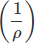In metals; when temperature increases, the velocity of free electrons increases and that leads to an increase in heat transfer and it also increases the collisions between the lattice ions and free electrons. This results in the drop in electrical conductivity.

The law defines the ratio of the electronic role of the thermal conductivity of a material to the electrical conductivity of a material (metal) is directly relative to the temperature.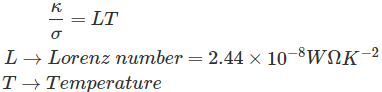This law is named after Gustav Wiedemann and Rudolph Franz in 1853 reported that the ratio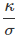has more or less the similar value for dissimilar metal at the same temperature.

## Derivation of the Law

For that, we have to assume a homogeneous isotropic material. This material is then subjected to a temperature gradient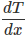. The direction of the heat flow will be opposite to that of the temperature gradient all the way through the conducting medium.
The heat flowing through the material per unit time per unit area is the heat flux. It will be proportional to the temperature gradient.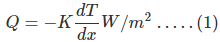K → Coefficient of thermal conductivity (W/mK)
K = Kphonon + Kelectron; since the transfer of heat in solids owing to phonon and electron.

Now, we can derive the expression for coefficient of thermal conductivity.
For that, we have to assume the flow of heat is from higher temperature to lower temperature in a metal slab which has a temperature gradient of.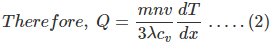cv → Specific heat
n → Number of particles per unit volume
λ → mean free path of collisions
v → velocity of electrons

Comparing the equations (1) and (2), we get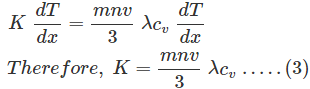We know that the energy of free electrons is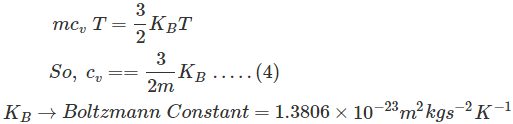We put the equation (4) in (3)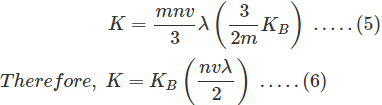Now, the specific heat for an ideal gas at constant volume,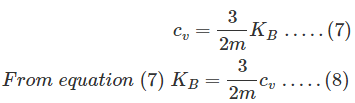When we put equation (8) in (6), we get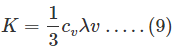Next, we can consider the electrical current density of a metal with the application of electric field, E (figure 1)
J = σ E ; Ohms law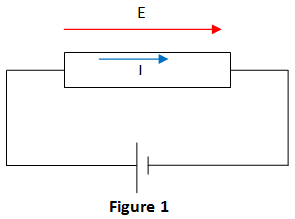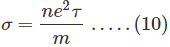So, the correct form of Ohms law is given by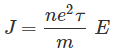There is a mean free path and mean time between the collisions.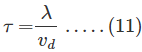e → Charge of the electron = 1.602 × 10-9 C
τ → Collision time or mean time: It is the average time for the electron to move or travel prior to scattering.
vdDrift Velocity: It is the standard velocity of the electron during the collision time.
When we put equation (11) in (10), we get electrical conductivity (Drude Conductivity) as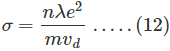Consider the electrons which move in a metal without any application of electrical field. Then the equipartition theorem is given by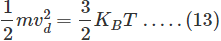From equation (13) we get m as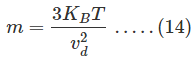Now, we put equation (14) in (12)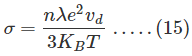Thus, we got the values of K and σ from equation (6) and (15).
Now, we can take the ratio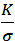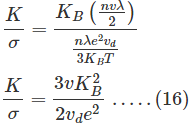We assume v = vd, then equation (16) becomes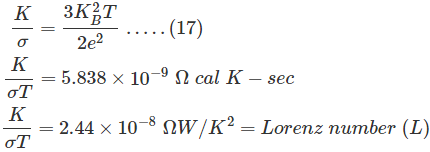From this, we can say that the ratiois similar for all the metals. It is also a function of temperature. This law is known as Wiedemann-Franz Lorenz Law. We can conclude that the most excellent electrical conductor will be a best thermal conductor.

## Limitations of Wiedemann Franz Law

• The value of L is not the same for all the materials.
• This law is not valid for intermediate temperature.
• In the pure metals, both σ and κ increases as temperature decreases.

Want To Learn Faster? 🎓
Get electrical articles delivered to your inbox every week.
No credit card required—it’s 100% free.